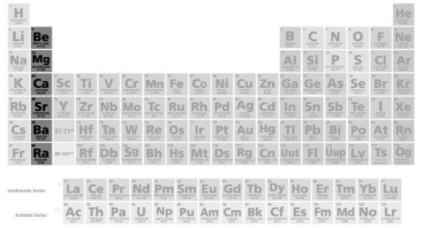QuestionAnswers

# The correct increasing order of solubility of sulphates in water is:A) ${\text{BeS}}{{\text{O}}_4}{\text{ }} > {\text{ MgS}}{{\text{O}}_4}{\text{ }} > {\text{ CaS}}{{\text{O}}_4}{\text{ }} > {\text{ SrS}}{{\text{O}}_4}{\text{ }} > {\text{ BaS}}{{\text{O}}_4}$B) ${\text{BaS}}{{\text{O}}_4}{\text{ }} > {\text{ SrS}}{{\text{O}}_4}{\text{ }} > {\text{ CaS}}{{\text{O}}_4}{\text{ }} > {\text{ MgS}}{{\text{O}}_4}{\text{ }} > {\text{ BeS}}{{\text{O}}_4}$C) ${\text{BaS}}{{\text{O}}_4}{\text{ }} > {\text{ CaS}}{{\text{O}}_4}{\text{ }} > {\text{ SrS}}{{\text{O}}_4}{\text{ }} > {\text{ BeS}}{{\text{O}}_4}{\text{ }} > {\text{ MgS}}{{\text{O}}_4}$D) ${\text{BaS}}{{\text{O}}_4}{\text{ }} > {\text{ SrS}}{{\text{O}}_4}{\text{ }} > {\text{ MgS}}{{\text{O}}_4}{\text{ }} > {\text{ CaS}}{{\text{O}}_4}{\text{ }} > {\text{ BeS}}{{\text{O}}_4}$Verified
128.7k+ views
Hint: Charge density and hydration energy are the two factors which mainly affect solubility of sulphates of alkaline earth metals in water.

Be, Mg, Ca, Sr and Ba are the elements of group 2 which are also known as alkaline earth metals. They are located in the second column of the periodic table.As we move from top to bottom in the group, the atomic and ionic radii increase. Solubility in water is related to the ionic nature and size and it generally decreases with increasing size.
So, the correct order of solubility of sulphates will be –
${\text{BeS}}{{\text{O}}_4}{\text{ }} > {\text{ MgS}}{{\text{O}}_4}{\text{ }} > {\text{ CaS}}{{\text{O}}_4}{\text{ }} > {\text{ SrS}}{{\text{O}}_4}{\text{ }} > {\text{ BaS}}{{\text{O}}_4}$
The small size, charge density and hydration energy favour beryllium sulphate and hence it has maximum solubility in water. However, in other sulphates, as the size increases, lattice energy increases and hydration energy decreases. So, solubility goes on decreasing.

Hence, option A is correct.

Additional Information: Ionic nature and size of the atom have a drastic impact on the solubility of alkaline earth metals in water. As the solubility decreases with increasing size, Beryllium ion has maximum stability whereas Barium ion has least solubility. Ions of smaller sizes tend to have higher charge density. Hence, they can be solvated by water molecules. This results in a higher enthalpy of hydration and makes the hydrated ions more stable.

Note: Solubility of alkaline earth metals increases with increase in atomic number. Hence, correct order of solubility of metal ions from maximum to minimum is ${\text{B}}{{\text{e}}^{ + 2}}{\text{ }} > {\text{ M}}{{\text{g}}^{ + 2}}{\text{ }} > {\text{ C}}{{\text{a}}^{ + 2}}{\text{ }} > {\text{ S}}{{\text{r}}^{ + 2}}{\text{ }} > {\text{ B}}{{\text{a}}^{ + 2}}$. This order is followed by most of the compounds of alkaline earth metals i.e. sulphates, carbonates etc.